Download Presentation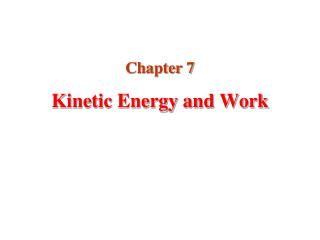Kinetic Energy and Work

# Kinetic Energy and Work - PowerPoint PPT Presentation

Kinetic Energy and Work. Chapter 7. Introduction to Energy. The concept of energy is one of the most important topics in science Every physical process that occurs in the Universe involves energy and energy transfers or transformations. Work and Energy.I am the owner, or an agent authorized to act on behalf of the owner, of the copyrighted work described.
Download Presentation## Kinetic Energy and Work

An Image/Link below is provided (as is) to download presentation

Download Policy: Content on the Website is provided to you AS IS for your information and personal use and may not be sold / licensed / shared on other websites without getting consent from its author.While downloading, if for some reason you are not able to download a presentation, the publisher may have deleted the file from their server.

- - - - - - - - - - - - - - - - - - - - - - - - - - E N D - - - - - - - - - - - - - - - - - - - - - - - - - -
Presentation Transcript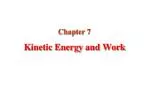### Kinetic Energy and Work

Chapter 7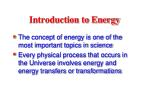Introduction to Energy
• The concept of energy is one of the most important topics in science
• Every physical process that occurs in the Universe involves energy and energy transfers or transformations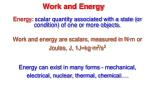Work and Energy

Energy:scalar quantity associated with a state (or condition) of one or more objects.

Work and energy are scalars, measured in N·m or Joules, J, 1J=kg∙m2/s2

Energy can exist in many forms - mechanical, electrical, nuclear, thermal, chemical….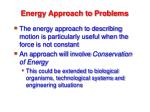Energy Approach to Problems
• The energy approach to describing motion is particularly useful when the force is not constant
• An approach will involve Conservation of Energy
• This could be extended to biological organisms, technological systems and engineering situations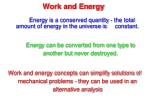Work and Energy

Energy is a conserved quantity - the total amount of energy in the universe is constant.

Energy can be converted from one type to another but never destroyed.

Work and energy concepts can simplify solutions of mechanical problems - they can be used in an alternative analysis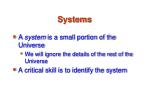Systems
• A system is a small portion of the Universe
• We will ignore the details of the rest of the Universe
• A critical skill is to identify the system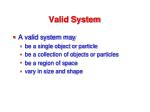Valid System
• A valid system may
• be a single object or particle
• be a collection of objects or particles
• be a region of space
• vary in size and shape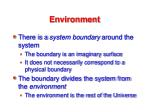Environment
• There is a system boundary around the system
• The boundary is an imaginary surface
• It does not necessarily correspond to a physical boundary
• The boundary divides the system from the environment
• The environment is the rest of the Universe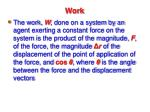Work
• The work,W, done on a system by an agent exerting a constant force on the system is the product of the magnitude,F, of the force, the magnitudeΔrof the displacement of the point of application of the force, andcos θ, whereθis the angle between the force and the displacement vectors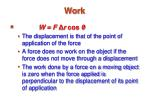Work
• W = FΔr cos θ
• The displacement is that of the point of application of the force
• A force does no work on the object if the force does not move through a displacement
• The work done by a force on a moving object is zero when the force applied is perpendicular to the displacement of its point of applicationWork Example
• The normal force,n, and the gravitational force,mg, do no work on the object

cos θ= cos 90° = 0

• The forceFdoes do work on the object• The system and the environment must be determined when dealing with work
• The environment does work on the system

(Work by the environment on the system)

• The sign of the work depends on the direction ofFrelative toΔr
• Work is positive when projection ofFontoΔris in the same direction as the displacement
• Work is negative when the projection is in the opposite directionWork Is An Energy Transfer
• This is important for a system approach to solving a problem
• If the work is done on a system and it is positive, energy is transferred to the system
• If the work done on the system is negative, energy is transferred from the systemWork done by a constant force

Work done on an object by a constant force is defined to be the product of the magnitude of the displacement and the component of the force parallel to the displacement

WhereFIIis the component of the forceFparallel to the displacementd

W = FII · dF

q

d

Work

W = F d cosq

In other words -

Whereθis the angle betweenFand d

Ifθis > 90o, work is negative. A decelerating car has negative work done on it by its engine.

The unit of work is called Joule (J), 1 J = 1 N·m

1J=kg∙m2/s2Scalar Product of Two Vectors
• The scalar product of two vectors is written asA . B

It is also called the dot product

• A . B = A B cos θ

θis the angle betweenAandBScalar Product
• The scalar product is commutative

A . B = B . A

• The scalar product obeys the distributive law of multiplication

A . (B + C) = A . B + A . CDot Products of Unit Vectors
• Using component form with AandB:Work - on and by

A person pushes block30 malong the ground by exerting force of25 Non the trolley. How much work does the person do on the trolley?

W = F d = 25N x 30m = 750 Nm

Trolley does -750 Nmwork on the person

Ftp

Fpt

dA force acts on an object as the object moves in the x direction from the origin to x = 5.00 m. Find the work W = Fdrdone on the object by the force.A force acts on an object as the object moves in the x direction from the origin to x = 5.00 m. Find the work W = Fdrdone on the object by the force.Mechanical Energy

Mechanical energy (energy associated with masses) can be thought of as having two components: kineticand potential

• Kinetic energy is energy of motion
• Potential energy is energy of positionKinetic Energy

In many situations, energy can be considered as“the ability to do work”

Energy can exist in different forms

Kinetic energy is the energy associated with the motion of an object

A moving object can do work on another object

• E.g hammer does work on nail.Kinetic Energy

Consider an object with massmmoving in a straight line with initial velocityvi. To accelerate it uniformly to a speedvfa constant net forceFis exerted on it parallel to motion over a distanced.

Work done on object

W = F d = m a d(NII)

SoKinetic Energy

If we rearrange this we obtain

We define the quantity½mv2to be the translational kinetic energy (KE) of the object

This is the ‘Work-Energy Theorem’:

“The net work done on an object is equal to its change in kinetic energy”

W = DKEThe Work-Energy Theorem

W = DKE

• Note
• The net work done on an object is the work done by the net force.
• Units of energy and work must be the same (J)Energy:scalar quantity associated with a state (or condition) of one or more objects.

• Kinetic energy.
• Work.
• Work - Kinetic energy theorem.
• Work done by a constant force
• - Gravitational force
• V. Work done by a variable force.
• - Spring force.
• 1D-Analysis
• 3D-Analysis
• VI. PowerI.Kinetic energy

Energy associated with the state of motion of an object.

Units:

1 Joule = 1J = 1 kgm2/s2

II. Work

To  +W

From  -W

Energy transferred “to” or “from” an object by means of a force acting on the object.- Constant force:

Work done by the force = Energy transfer due to the force.To calculate the work done on an object by a force during

• a displacement, we use only the force component along
• the object’s displacement. The force component perpendicular
• to the displacement does zero work.
• Assumptions:1) F = constant force
• 2) Object is particle-like (rigid object, all parts of the object must move together).

A force does+Wwhen it has a vector component in the same direction as the displacement, and–Wwhen it has

a vector component in the opposite direction.W=0when it has no such vector component.Net work done by several forces = Sum of works done by

individual forces.

Calculation:1) Wnet= W1+W2+W3+…

2) Fnet  Wnet=Fnet dII. Work-Kinetic Energy Theorem

Change in the kinetic energy of the

particle = Net work done on the particle

III. Work done by a constant force

- Gravitational force:

Rising object:W= mgd cos180º = -mgd

Fgtransfers mgdenergy from the object’s kinetic energy.

Falling down object:W= mgd cos 0º = +mgd

Fgtransfers mgdenergy to the object’s kinetic energy.External applied force + Gravitational force:

Object stationary before and after the lift:Wa+Wg=0

The applied force transfers the same amount of energy to the object as the gravitational force transfers from the object.IV. Work done by a variable force

- Spring force:

Hooke’s law

k= spring constant  measures spring’s stiffness.Units:N/mHooke’s Law
• When xis positive (spring is stretched),Fis negative
• Whenx is0(at the equilibrium position),F is0
• When xis negative (spring is compressed),Fis positiveHooke’s Law
• The force exerted by the spring is always directed opposite to the displacement from equilibrium
• Fis called the restoring force
• If the block is released it will oscillate back and forth between –x and xHooke’s law:

xi

Δx

x

Work done by a spring force:

• Spring is massless mspring << mblock
• Ideal spring  obeys Hooke’s law exactly.
• Contact between the block and floor is frictionless.
• Block is particle-like.

Assumptions:- Calculation:

• The block displacement must be divided
• into many segments of infinitesimal width,Δx.
• 2)F(x) ≈ ctewithin each shortΔxsegment.

Fx

xi

Δx

xf

x

Fj

Ws>0  Block ends up closer to the relaxed position (x=0) than it was initially.

Ws<0  Block ends up further away fromx=0.

Ws=0  Block ends up at x=0.Work done by an applied force + spring force:

Block stationary before and after the displacement:

Wa= -Ws The work done by the applied force displacing

the block is the negative of the work done by the spring force.If it takes4.00 Jof work to stretch a Hooke's-law spring10.0 cmfrom its unstressed length, determine the extra work required to stretch it an additional10.0 cm.If it takes4.00 Jof work to stretch a Hooke's-law spring10.0 cmfrom its unstressed length, determine the extra work required to stretch it an additional10.0 cm.A2.00-kgblock is attached to a spring of force constant500 N/m.The block is pulled5.00 cmto the right of equilibrium and released from rest. Find the speed of the block as it passes through equilibrium if (a) the horizontal surface is frictionless and (b) the coefficient of friction between block and surface is0.350.A2.00-kgblock is attached to a spring of force constant500 N/m.The block is pulled5.00 cmto the right of equilibrium and released from rest. Find the speed of the block as it passes through equilibrium if (a) the horizontal surface is frictionless and (b) the coefficient of friction between block and surface is0.350.A2.00-kgblock is attached to a spring of force constant500 N/m.The block is pulled5.00 cmto the right of equilibrium and released from rest. Find the speed of the block as it passes through equilibrium if (a) the horizontal surface is frictionless and (b) the coefficient of friction between block and surface is0.350.Work done by a general variable force:
• Assume that during a very small displacement,Δx,Fis constant
• For that displacement,W ~ FΔx
• For all of the intervals,Work done by a general variable force:
• Therefore,
• The work done is equal to the area under the curve3D-Analysis

Work-Kinetic Energy Theorem - Variable forceV. Power

Time rate at which the applied force does work.

- Average power:amount of work done

in an amount of time Δt by a force.

• Instantaneous power:
• instantaneous time rate of doing work.

Units:1 watt= 1 W = 1J/s

1 kilowatt-hour = 1000W·h = 1000J/s x 3600s = 3.6 x 106 J

= 3.6 MJ

F

φ

xN

Fx

mg

Fy

In the figure below a2N force is applied to a4kgblock at a

downward angleθas the block moves right-ward through1macross a frictionless floor. Find an expression for the speedvfat the end of that distance if the block’s initial velocity is: (a)0and (b)1m/sto the right.

(a)

N

Fx

Fy

mgIn the figure below a2N force is applied to a4kgblock at a

downward angleθas the block moves right-ward through1macross a frictionless floor. Find an expression for the speedvfat the end of that distance if the block’s initial velocity is: (a)0and (b)1m/sto the right.

N

Fx

mg

Fy

(a)

N

Fx

Fy

mg(c) The situation in fig.(b) is similar in that the block is initially moving at1m/sto the right, but now the2Nforce is directed downward to the left. Find an expression for the speed of the block at the end of the 1m distance.

N

Fx

mg

Fy

N

Fx

Fy

mg(c) The situation in fig.(b) is similar in that the block is initially moving at1m/sto the right, but now the2Nforce is directed downward to the left. Find an expression for the speed of the block at the end of the 1m distance.

N

Fx

mg

Fy

N

Fx

Fy

mgA small particle of mass mis pulled to the top of a frictionless half-cylinder (of radius R) by a cord that passes over the top of the cylinder, as illustrated in Figure. (a) If the particle moves at a constant speed, show that F = mgcos. (Note: If the particle moves at constant speed, the component of its acceleration tangent to the cylinder must be zero at all times.) (b) By directly integrating W = Fdr, find the work done in moving the particle at constant speed from the bottom to the top of the half-cylinder.A small particle of mass mis pulled to the top of a frictionless half-cylinder (of radius R) by a cord that passes over the top of the cylinder, as illustrated in Figure. (a) If the particle moves at a constant speed, show that F = mgcos. (Note: If the particle moves at constant speed, the component of its acceleration tangent to the cylinder must be zero at all times.) (b) By directly integrating W = Fdr, find the work done in moving the particle at constant speed from the bottom to the top of the half-cylinder.

(a)A small particle of mass mis pulled to the top of a frictionless half-cylinder (of radius R) by a cord that passes over the top of the cylinder, as illustrated in Figure. (a) If the particle moves at a constant speed, show that F = mgcos. (Note: If the particle moves at constant speed, the component of its acceleration tangent to the cylinder must be zero at all times.) (b) By directly integrating W = Fdr, find the work done in moving the particle at constant speed from the bottom to the top of the half-cylinder.

(b)

(We use radian measure to express the next bit of displacement dr as in terms of the next bit of angle moved through dθ: dr=Rdθ)Two springs with negligible masses, one with spring constant k1 and the other with spring constant k2, are attached to the endstops of a level air track as in Figure. A glider attached to both springs is located between them. When the glider is in equilibrium, spring 1 is stretched by extension xi1 to the right of its unstretched length and spring 2 is stretched by xi2 to the left. Now a horizontal force Fapp is applied to the glider to move it a distance xa to the right from its equilibrium position. Show that in this process (a) the work done on spring 1 is k1(xa2+2xaxi1) , (b) the work done on spring 2 is k2(xa2 – 2xaxi2) , (c) xi2 is related to xi1 by xi2 = k1xi1/k2, and (d) the total work done by the force Fapp is (k1 + k2)xa2.Two springs with negligible masses, one with spring constant k1 and the other with spring constant k2, are attached to the endstops of a level air track as in Figure. A glider attached to both springs is located between them. When the glider is in equilibrium, spring 1 is stretched by extension xi1 to the right of its unstretched length and spring 2 is stretched by xi2 to the left. Now a horizontal force Fapp is applied to the glider to move it a distance xa to the right from its equilibrium position. Show that in this process (a) the work done on spring 1 is k1(xa2+2xaxi1) , (b) the work done on spring 2 is k2(xa2 – 2xaxi2) , (c) xi2 is related to xi1 by xi2 = k1xi1/k2, and (d) the total work done by the force Fapp is (k1 + k2)xa2.

(a)

(b)Two springs with negligible masses, one with spring constant k1 and the other with spring constant k2, are attached to the endstops of a level air track as in Figure. A glider attached to both springs is located between them. When the glider is in equilibrium, spring 1 is stretched by extension xi1 to the right of its unstretched length and spring 2 is stretched by xi2 to the left. Now a horizontal force Fapp is applied to the glider to move it a distance xa to the right from its equilibrium position. Show that in this process (a) the work done on spring 1 is k1(xa2+2xaxi1) , (b) the work done on spring 2 is k2(xa2 – 2xaxi2) , (c) xi2 is related to xi1 by xi2 = k1xi1/k2, and (d) the total work done by the force Fapp is (k1 + k2)xa2.

(c)

Before the horizontal force is applied, the springs exert equal forces:Two springs with negligible masses, one with spring constant k1 and the other with spring constant k2, are attached to the endstops of a level air track as in Figure. A glider attached to both springs is located between them. When the glider is in equilibrium, spring 1 is stretched by extension xi1 to the right of its unstretched length and spring 2 is stretched by xi2 to the left. Now a horizontal force Fapp is applied to the glider to move it a distance xa to the right from its equilibrium position. Show that in this process (a) the work done on spring 1 is k1(xa2+2xaxi1) , (b) the work done on spring 2 is k2(xa2 – 2xaxi2) , (c) xi2 is related to xi1 by xi2 = k1xi1/k2, and (d) the total work done by the force Fapp is (k1 + k2)xa2.

(d)N6.A2kglunchbox is sent sliding over a frictionless surface, in the positive direction of anxaxis along the surface. Beginning att=0, a steady wind pushes on the lunchbox in the negative direction ofx. Estimate the kinetic energy of the lunchbox at (a) t=1s, (b)t=5s. (c) How much work does the force from the wind do on the lunch box fromt=1s tot=5s?N6.A2kglunchbox is sent sliding over a frictionless surface, in the positive direction of anxaxis along the surface. Beginning att=0, a steady wind pushes on the lunchbox in the negative direction ofx. Estimate the kinetic energy of the lunchbox at (a) t=1s, (b)t=5s. (c) How much work does the force from the wind do on the lunch box fromt=1s tot=5s?the positive direction of an x axis along the surface. Beginning

at t=0, a steady wind pushes on the lunchbox in the negative

direction of x, Fig. below. Estimate the kinetic energy of the

lunchbox at (a) t=1s, (b) t=5s. (c) How much work does the force

from the wind do on the lunch box from t=1s to t=5s?N

Fgx

Fgy

mg

N12.In the figure below a horizontal forceFaof magnitude20N is applied to a3kgbook, as the book slides a distance ofd=0.5m up a frictionless ramp. (a) During the displacement, what is the net force done on the book byFa, the gravitational force on the book and the normal force on the book? (b) If the book has zero kinetic energy at the start of the displacement, what is the speed at the end of the displacement?

x

yN12.In the figure below a horizontal forceFaof magnitude20N

is applied to a3kgbook, as the book slides a distance ofd=0.5m

up a frictionless ramp. (a) During the

displacement, what is the net force done

on the book byFa, the gravitational force

on the book and the normal force on the

book? (b) If the book has zero kinetic

energy at the start of the displacement,

what is the speed at the end of the

displacement?

N

Fgx

Fgy

mg

y

xis applied to a 3kg book, as the book slides a distance of d=0.5m

up a frictionless ramp. (a) During the

displacement, what is the net force done

on the book by Fa, the gravitational force

on the book and the normal force on the

book? (b) If the book has zero kinetic

energy at the start of the displacement,

what is the speed at the end of the

displacement?

N

Fgx

Fgy

mg

y

xin displacing the particle from x=1 to x=3m. (b) The curve is given

by F=a/x2, with a=9Nm2. Calculate the work using integrationin displacing the particle from x=1 to x=3m. (b) The curve is given

by F=a/x2, with a=9Nm2. Calculate the work using integrationload of 1800kg. If the cab is moving upward at full load at constant

speed 3.8m/s, what power is required of the force moving the cab

to maintain that speed?

Fa

mgload of 1800kg. If the cab is moving upward at full load at constant

speed 3.8m/s, what power is required of the force moving the cab

to maintain that speed?

Fa

mgThe figure below shows the velocity component versus time for

the body. For each of the intervals

AB, BC, CD, and DE, give the sign

(plus or minus) of the work done by

the force, or state that the work is zero.

v

B

C

D

A

t

EThe figure below shows the velocity component versus time for

the body. For each of the intervals

AB, BC, CD, and DE, give the sign

(plus or minus) of the work done by

the force, or state that the work is zero.

v

B

C

D

A

t

E15E.In the figure below, a cord runs around two massless,

frictionless pulleys; a canister with mass m=20kghangs from one

pulley; and you exert a force Fon the free end of the cord.

(a) What must be the magnitude of Fif

you are to lift the canister at a constant

speed? (b) To lift the canister by 2cm,

how far must you pull the free end of

the cord? During that lift, what is the

work done on the canister by (c) your

force (via the cord) and (d) the

gravitational force on the canister?

P2

T

T

T

P1

mg15E.In the figure below, a cord runs around two massless,

frictionless pulleys; a canister with mass m=20kghangs from one

pulley; and you exert a force Fon the free end of the cord.

(a) What must be the magnitude of Fif

you are to lift the canister at a constant

speed? (b) To lift the canister by 2cm,

how far must you pull the free end of

the cord? During that lift, what is the

work done on the canister by (c) your

force (via the cord) and (d) the

gravitational force on the canister?

P2

T

T

T

P1

mg15E.In the figure below, a cord runs around two massless,

frictionless pulleys; a canister with mass m=20kg hangs from one

pulley; and you exert a force F on the free end of the cord.

(a) What must be the magnitude of F if

you are to lift the canister at a constant

speed? (b) To lift the canister by 2cm,

how far must you pull the free end of

the cord? During that lift, what is the

work done on the canister by (c) your

force (via the cord) and (d) the

gravitational force on the canister?

P2

T

T

T

P1

mg

(b) To rise “m”0.02m, two segments of the cord must be shorten by that amount. Thus, the amount of the string pulled down at the left end is: 0.04m15E.In the figure below, a cord runs around two massless,

frictionless pulleys; a canister with mass m=20kg hangs from one

pulley; and you exert a force F on the free end of the cord.

(a) What must be the magnitude of F if

you are to lift the canister at a constant

speed? (b) To lift the canister by 2cm,

how far must you pull the free end of

the cord? During that lift, what is the

work done on the canister by (c) your

force (via the cord) and (d) the

gravitational force on the canister?

P2

T

T

T

P1

mg

WF+WFg=0

There is no change in kinetic energy.Challenging problems – Chapter 7

Two trolleys of masses m1=400 kg and m2=200 kg are connected by a rigid rod. The trolleys lie on a horizontal frictionless floor. A man wishes to push them with a force of 1200N.

From the point of view of kinetics,

does the relative position of the

trolleys matter? If the rod can

only stand an applied force of 500N,

which trolley should be up front?

N1

N2

Situation 1

F2r

F1r

F

m1g

m2gChallenging problems – Chapter 7

Two trolleys of masses m1=400 kg and m2=200 kg are connected by a rigid rod. The trolleys lie on a horizontal frictionless floor. A man wishes to push them with a force of 1200N.

From the point of view of kinetics,

does the relative position of the

trolleys matter? If the rod can

only stand an applied force of 500N,

which trolley should be up front?

N1

N2

Situation 1

F2r

F1r

F

m1g

m2g

Action and reaction forces: F1r force that the rod does on m1

F2r force that the rod does on m2

F’2r

F’1r

F’1r force that m1 does on rod.

F’2r force that m2 does on rod.

Situation 1

Rod negiglible mass, Fnet on rod=0

F’1r = F’2r  rigid rod does not deform

F1r = F’1r  Action / Reaction

Forces that the rod does on the blocks as it tries to counteract the deformation that F could induce.Challenging problems – Chapter 7

Two trolleys of masses m1=400 kg and m2=200 kg are connected by a rigid rod. The trolleys lie on a horizontal frictionless floor. A man wishes to push them with a force of 1200N. From the point of view of kinetics, does the relative position of the trolleys matter? If the rod can only stand an applied force of 500N, which trolley should be up front?

F’2r

F’1r

Action and reaction forces: F1r force that the rod does on m1

F2r force that the rod does on m2

Situation 2

F’1r force that m1 does on rod.

F’2r force that m2 does on rod.

Rod negiglible mass, Fnet on rod=0

F’1r = F’2r  rigid rod does not deform

F1r = F’1r  Action / Reaction

Situation 2

N2

N1

From kinetic point of view, Situation1=Situation2same acceleration”.

F1r

F2r

F

From dynamics  F1r(1)≠F1r(2) Only situation (1) is possible F1r=F’1r = 400N <Fmax=500N

m1g

m2g2.A car with a weight of 2500N working with a power of 130kW develops a velocity of 112 km/hour when traveling along a horizontal straight highway. Assuming that the frictional forces (from the ground and air) acting on the car are constant but not negligible: What is the value of the frictional forces?

(a) What is the car’s maximum velocity on a 50incline hill? (b) What is the power if the car is traveling on a 100 inclined hill at 36km/h?2.A car with a weight of 2500N working with a power of 130kW develops a velocity of 112 km/hour when traveling along a horizontal straight highway. Assuming that the frictional forces (from the ground and air) acting on the car are constant but not negligible: What is the value of the frictional forces?

(a) What is the car’s maximum velocity on a 50 incline hill? (b) What is the power if the car is traveling on a 100 inclined hill at 36km/h?

N

f

F

Situation 1

mg2.A car with a weight of 2500N working with a power of 130kW develops a velocity of 112 km/hour when traveling along a horizontal straight highway. Assuming that the frictional forces (from the ground and air) acting on the car are constant but not negligible: (a) What is the car’s maximum velocity on a 50 incline hill? (b) What is the power if the car is traveling on a 100 inclined hill at 36km/h?

Situation 2

N

F

Fgx

Fgy

f

mg